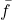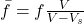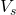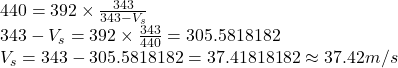A car speeding down the highway honks its horn, which has a frequency 392 Hz, but a resting bystander hears the frequency 440 Hz. If the spe

Question

A car speeding down the highway honks its horn, which has a frequency 392 Hz, but a resting bystander hears the frequency 440 Hz. If the speed of sound in air is 343 m/s, how fast is the car moving

in progress 0
5 months 2021-09-02T15:22:44+00:00 1 Answers 1 views 0

We know that apparent frequency,is given bywhere f is the given frequency in this case 392, V is the speed of sound in air which is given as 343 andis the speed of car which is unknown, \bar f is given as 440 Hz